# Torque Equation of  Three Phase Induction Motor:

The torque (T) is developed in a three phase induction motor depends upon the following three factors:

1. As First, the rotating magnetic field which is responsible to produce Induced EMF in the rotor(φ)
2. Magnitude of rotor current in motor running condition (I2),
3. The power factor of rotor of the three phase induction motor (cosθ2).

With all relationship we can expressed as mathematical term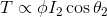Where,

T is the torque produced by induction motor,

φ is magnetic flux ,

I2 is rotor running current,

cosθ2 is the power factor of rotor during running condition.

The flux φ produced by the stator is proportional to stator emf supply voltage E1.

As we know the Induction motor works as stepdown transformer. Turns ratio K is defined as the ratio between the secondary voltage (rotor voltage) to primary voltage (stator voltage).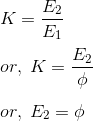Rotor current I2 is defined as the ratio of rotor induced emf under running condition ,

I2= Rotor EMF/ Total Impedance

I2=sE2/Z2

But Total Impedance Z2 is equal to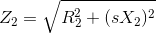sub in Rotor current I2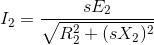Here s = slip of Induction motor

We know that power factor is defined as ratio of resistance to that of impedance. The power factor of the rotor circuit is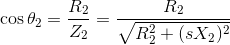Putting the value of flux φ, rotor current I2, power factor cosθ2 in the equation of torque we get,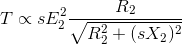Combining similar term we get, Removing proportionality constant then we get..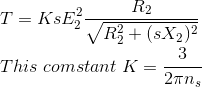Where, ns is synchronous speed in rps (revolution per second) ns = Ns / 60. So, finally the equation of torque becomes,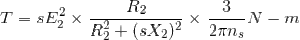This is Torque Equation of Three Phase Induction Motor

## Conclusion:

As per this Torque equation, the developing torque in Induction Motor is directly proportional to  the square of the applied voltage.